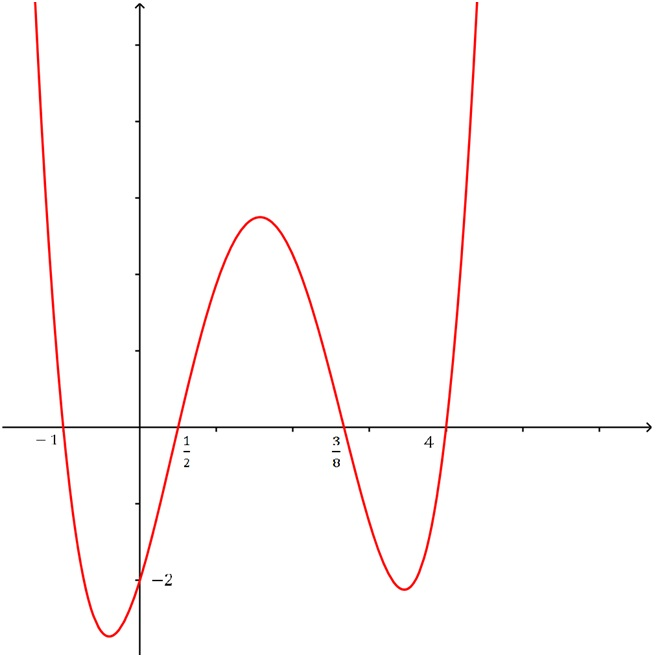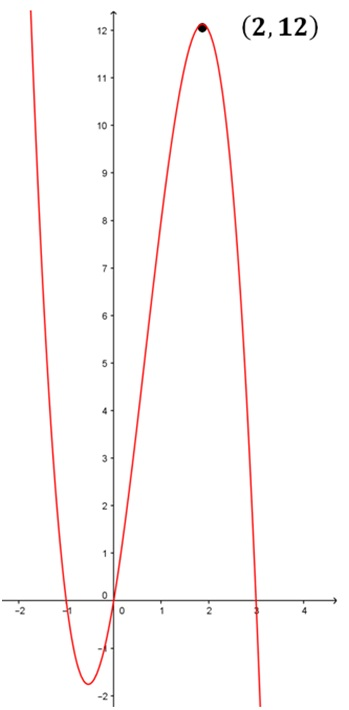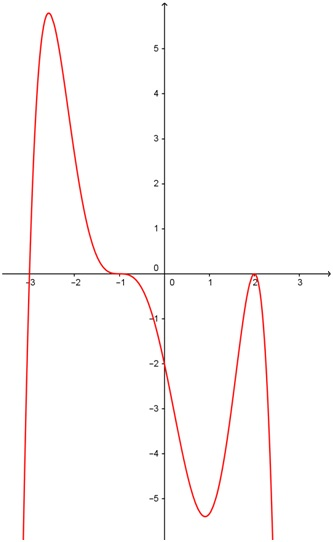# Determining the equation of a polynomial function#### Everything You Need in One Place

Homework problems? Exam preparation? Trying to grasp a concept or just brushing up the basics? Our extensive help & practice library have got you covered.#### Learn and Practice With Ease

Our proven video lessons ease you through problems quickly, and you get tonnes of friendly practice on questions that trip students up on tests and finals.#### Instant and Unlimited Help

Our personalized learning platform enables you to instantly find the exact walkthrough to your specific type of question. Activate unlimited help now!##### Intros
###### Lessons
1. Steps to Finding the Equation of a Polynomial Function
##### Examples
###### Lessons
1. Determining the Equation of a Polynomial Function With Multiplicities Equal to 1
Determine the equation of the polynomial function of lowest possible degree in factored form.
1.2.2. Determining the Equation of a Polynomial Function With Higher Multiplicities
Determine the equation of the polynomial function of lowest possible degree in factored form.###### Topic Notes
We can easily find the equation of a polynomial from its graph by identifying x-intercept and the sign of the leading coefficient. That way, we can determine the factors of the polynomial and the end behavior of the function.
Steps to determining the equation of a polynomial function
1. Find the zeros, write them out in factored form.
2. Determine the multiplicity each zero by observing the behavior of the graph near the zero.
3. Determine the leading coefficient C by plugging in the coordinates of a point (other than the x-intercepts) on the graph.